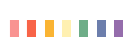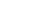# 单利与复利

## 单利

I=P×r×n 利息额与本金、利率、时间呈线性关系

I2=100×6%×2=12

I3=100×6%×3=18……

## 复利

1、一年付息一次(一年复利一次)

I=S-P=P(1+r)n-P=P[(1+r)n-1]

I1=S1-P1=100(1+6%)-100=6

I2=S2-P2=100(1+6%)2-100=12.36>12

I3=S3-P3=100(1+6%)3-100=19.10>18……

I为利息额。利息额与利率、时间呈非线性关系(只与本金呈线性关系)

2、一年付息多次(一年复利多次)

6个月末的终值为：FV=100×(1+0.06/2)=103

## 连续复利

 分类角度 分类类别 利率的决定方式 固定利率 浮动利率 利率的真实水平 名义利率 实际利率 借贷主体 中央银行利率（再贴现利率、再贷款利率） 商业银行利率（存款利率、贷款利率、贴现率） 非银行利率（债券利率、企业利率、金融利率） 计算利率的期限单位 年利率 年利率=月利率×12=日利率×360 月利率 日利率

• 每年计息次数越多，终值越大；
• 随计息间隔的缩短(计息次数的增加)，终值以递减速度增加，最后等于连续复利的终值。• QQ咨询
• 回顶